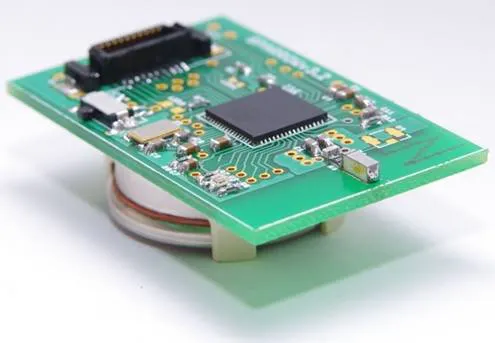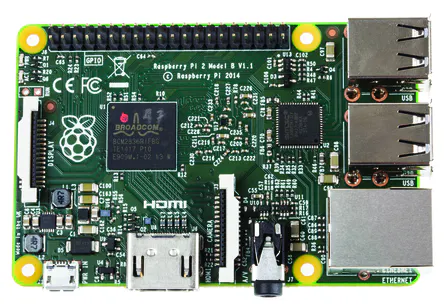Published © GPL3+

# Using Sparrow v3 for indoor and outdoor monitoring

Using Sparrow v3 and DeviceHub.net for indoor and outdoor wireless sensor node monitoring

IntermediateFull instructions provided2,740## Things used in this project

### Hardware components

 Sparrow Wireless Sensor Node Link to store coming soon ...
×1Raspberry Pi 2 Model B Raspberry Pi 1 would fit as well.
×1

### Software apps and online servicesArduino IDEDeviceHub.net DeviceHub Cloud Service - Standard package

Read more

## Code

### Simple test program

C/C++
Blinks a led on Sparrow. Its purpose is to check that Sparrow support was correctly set in Arduino IDE.
```#include <util/delay.h>

int main(void)
{
// Serial needs interrupts.
sei();

DDRB = (1<<PB5);
PORTB = 0x00;

// Print a message on the Serial interface
Serial.begin(9600);
Serial.println("Demo of how to use Serial and blink LEDs");
Serial.end();

// Make the LED blink
while(1)
{
// Change the LED's state
PORTB ^= (1<<PB5);
// Wait 1 sec
_delay_ms(1000);
}

return 0;
}
```

### Arduino code - low power monitoring project.

C/C++
Common code for monitoring nodes and gateway node. Differentiation between these types of nodes is made starting from the node id.
```#include <avr/io.h>
#include <avr/sleep.h>
#include <avr/interrupt.h>
#define F_CPU 16000000UL
#include <util/delay.h>
#include <stdio.h>
#include "sht21.h"

#define _DEBUG_ 0

#define TRX_FRAME_BUFFER(index) (*(volatile uint8_t *)(0x180 + (index)))

// Modify these addresses accordingly before uploading the code on each node
uint8_t nod1_address __attribute__((section(".data"))) = 1;
uint8_t node_address __attribute__((section(".data"))) = 1;

short light, voltage;
float temp, humid;
byte seq = 0;

// Interrupt triggered when the Symbol Counter reaches to 0.
ISR(SCNT_CMP1_vect)
{
//Do nothing
}

uint32_t symbol_threshold = 0x00000000;
void initializeSymbolCounter()
{
// enable asynchronous mode, with external oscillator (32.768kHz in our case)
ASSR |= _BV(AS2);

SCOCR1HH = (symbol_threshold >> 24);
SCOCR1HL = (symbol_threshold & 0x00ff0000) >> 16;
SCOCR1LH = (symbol_threshold & 0x0000ff00) >>  8;
SCOCR1LL = (symbol_threshold & 0x000000ff);
SCCR0 = _BV(SCEN);
SCCNTHH = 0x00;
SCCNTHL = 0x00;
SCCNTLH = 0x00;
SCCNTLL = 0x00;

while (SCSR & _BV(SCBSY));
// enable compare match 1 IRQ
SCIRQM = _BV(IRQMCP1);
}

void delaySymbolCounter(uint8_t seconds)
{
symbol_threshold += (((uint32_t)seconds) * 62500);

SCOCR1HH = (symbol_threshold >> 24);
SCOCR1HL = (symbol_threshold & 0x00ff0000) >> 16;
SCOCR1LH = (symbol_threshold & 0x0000ff00) >>  8;
SCOCR1LL = (symbol_threshold & 0x000000ff);

while (SCSR & _BV(SCBSY));
}

void setState(uint8_t state)
{
TRX_STATE = CMD_FORCE_TRX_OFF;
TRX_STATE = state;
while (state != TRX_STATUS_struct.trx_status);
}

// send a short frame with: current node id and the values read from the sensors.
void sendFrame()
{
setState(CMD_PLL_ON);

TRX_FRAME_BUFFER(0) = 9;	//length - minimum length is 3
TRX_FRAME_BUFFER(1) = node_address;
TRX_FRAME_BUFFER(2) = seq++;
TRX_FRAME_BUFFER(3) = (byte)temp;
TRX_FRAME_BUFFER(4) = (byte)humid;
TRX_FRAME_BUFFER(5) = (byte)light;
TRX_FRAME_BUFFER(6) = (byte)voltage;

// start transmission
TRX_STATE = CMD_TX_START;
}

volatile byte receivedId = 0;
volatile byte receivedS;
volatile byte receivedT;
volatile byte receivedH;
volatile byte receivedL;
volatile byte receivedV;

// Interrupt triggered after detecting the end of a transmission
// Here we parse the received data
ISR(TRX24_RX_END_vect)
{
receivedId = TRX_FRAME_BUFFER(0);

PORTB ^= (1<<PB5);

receivedS = TRX_FRAME_BUFFER(1);
receivedT = TRX_FRAME_BUFFER(2);
receivedH = TRX_FRAME_BUFFER(3);
receivedL = TRX_FRAME_BUFFER(4);
receivedV = TRX_FRAME_BUFFER(5);

setState(CMD_RX_ON);
}

void rfInit(void)
{
setState(CMD_TRX_OFF);
IRQ_STATUS = 0xff;
IRQ_MASK_struct.rx_end_en = 1;
}

int main(void)
{
sei();

if (node_address == 0)
{
DDRB |= _BV(PD0);
PORTB = 0x00;
}
else
{
DDRE = 0xff;
PORTE = 0xff;
PRR0 &= ~_BV(PRADC);
initializeSymbolCounter();
}

rfInit();

// Init UART for node 0 (the gateway)
if (node_address == 0)
Serial.begin(9600);

while(1)
{
// Node 0 is the gateway
if (node_address != 0)
{
// Set symbol counter for 1 second
delaySymbolCounter(1);

// Start sensors
DDRE |= _BV(PE7);
PORTE &= ~_BV(PE7);

// No power reduction for ADC
PRR0 &= ~_BV(PRADC);

// Init twi
twiInit();

// Wait for sensor startup
_delay_ms(10);

// Read data from sht21 sensor (humid and temp)
uint16_t value16temp = SHTReadValue(0xE3);
TWCR = 0;
uint16_t value16humid = SHTReadValue(0xE5);
TWCR = 0;

// Turn sensors off
DDRE &= ~_BV(PE7);
PORTE &= ~_BV(PE7);

// Adjust sht21 readed values
temp = ((float)value16temp) / 374.23 - 46.85;
humid = ((float) value16humid) / 524.288 - 6;

// Read voltage value from PF0. For Atmega128RFA1 PF0 is an input for ADC0
// Enable ADC and set ADC prescalar to 128 - 125KHz sample rate @ 16MHz
ADCSRA = _BV(ADEN) | _BV(ADPS0) | _BV(ADPS2) | _BV(ADPS1);
// Left adjust ADC result to allow easy 8 bit reading and Set ADC reference to AVCC
ADMUX = _BV(ADLAR) | _BV(REFS0);
// Poll to see if AVDD has been powered-up.
loop_until_bit_is_set(ADCSRB, AVDDOK);
// Start the conversion
ADCSRA |= _BV(ADSC);
// Poll to see if A/D conversion is completed
loop_until_bit_is_set(ADCSRA, ADIF);
voltage = ADCH;

// Read light value from PF2. For Atmega128RFA1 PF2 is an input for ADC2
// Left adjust ADC result to allow easy 8 bit reading; Set ADC reference to AVCC; Select the input from ADC2
ADMUX = _BV(ADLAR) | _BV(REFS0) | _BV(MUX1);
// Poll to see if AVDD has been powered-up.
loop_until_bit_is_set(ADCSRB, AVDDOK);
// Start the conversion
ADCSRA |= _BV(ADSC);
// Poll to see if A/D conversion is completed
loop_until_bit_is_set(ADCSRA, ADIF);
light = ADCH;

// TWI and ADC power reduction; reset ADCSRA
ADCSRA = 0;
PRR0 |= _BV(PRADC);
PRR0 |= _BV(PRTWI);

#if _DEBUG_
// Send data on the serial port. Useful for debugging non-gateway nodes
// NOTE: I observed that you can't use a single Serial.begin, at the beggining of main function,
// and reuse that Serial after waking up from sleep. Junk is printed on the serial in that case.
// So I use a Serial.begin each time I want to print something and a Serial.end after I finished.
Serial.begin(9600);
Serial.print("humid, temp, light, voltage: ");
Serial.print((byte)humid);
Serial.print(", ");
Serial.print((byte)temp);
Serial.print(", ");
Serial.print((byte)light);
Serial.print(", ");
Serial.println((byte)voltage);
Serial.flush();
Serial.end();
#endif

// Wake transceiver; Send data through the transceiver to a listening gateway
TRXPR &= ~_BV(SLPTR);
sendFrame();

// Wait for transmisson complete
while(TRX_STATUS_struct.trx_status == BUSY_TX);
_delay_us(100);

// Put transceiver down
setState(CMD_TRX_OFF);
TRXPR = 1 << SLPTR;

// Sleep and wait to be waken when the symbol counter interrupt is triggered
set_sleep_mode(SLEEP_MODE_PWR_SAVE);
sleep_enable();
sleep_cpu();
sleep_disable();
}
else
{
setState(CMD_RX_ON);
// Do nothing while you have not received a new data package
while (receivedId == 0) asm("nop");

//send data on the serial port
Serial.print(receivedId);
Serial.print(":");
Serial.print(TST_RX_LENGTH_struct.rx_length);
Serial.print(":");
Serial.print(receivedS);
Serial.print(":");
Serial.print(receivedT);
Serial.print(":");
Serial.print(receivedL);
Serial.print(":");
Serial.print(receivedH);
Serial.print(":");
Serial.println(receivedV);

// Blink a Led, for debugging purposes
PORTB ^= (1<<PB5);

// Reset receivedId
receivedId = 0;
}
}
}
```

### Devicehub script.

Python
Running on the Raspberry Pi.
```#!/usr/bin/env python

__author__ = 'Alex Marin'
import random
import time
from time import sleep

from devicehub.devicehub import Sensor, Actuator, Device, Project

import serial

ser = serial.Serial('COM8', 9600, timeout=36000)

PROJECT_ID = '2145'
DEVICE_UUID = '7cz06af3-4427-4085-aeb0-22weq30f268e'
API_KEY = '08a99870-4a47-4578-b290-4e49c4102e88'

project = Project(PROJECT_ID, persistent=False)
device = Device(project, DEVICE_UUID, API_KEY)

temp_s = Sensor(Sensor.ANALOG, 'temp')
light_s = Sensor(Sensor.ANALOG, 'light')
rh_s = Sensor(Sensor.ANALOG, 'rh')
battery_s = Sensor(Sensor.ANALOG, 'voltage')

device.addSensor(temp_s)
device.addSensor(light_s)
device.addSensor(rh_s)
device.addSensor(battery_s)

while 1:
line = ser.readline()

id_v = int(line.split(':'))
temp_v = int(line.split(':'))
light_v = int(line.split(':'))
rh_v = int(line.split(':'))
battery_v = int(line.split(':'))

print "id:",id_v," temp:",temp_v," light:",light_v," rh:",rh_v," battery:",float(battery_v/71.0)

temp_s.addValue(temp_v)
light_s.addValue(light_v)
rh_s.addValue(rh_v)
battery_s.addValue(float(battery_v/71.0))

device.send()
```

## Credits

### Marin Alexandru-Gabriel

1 project • 5 followers

### Dan Tudose

5 projects • 28 followers

### Andrei Voinescu

1 project • 5 followers
PhD student on Wireless Sensor Networks, University POLITEHNICA of Bucharest